/

### The log-logistic distribution

 Distribution name Log-logistic distribution Common notation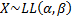Parameters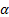= scale parameter (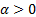)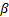= shape parameter (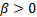) Domain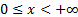Probability density function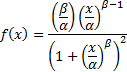Cumulative distribution function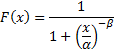Mean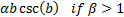where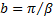and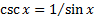is the cosecant function Variance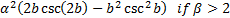Other comments Is also called the Fisk distribution. Its non-central moments are (if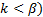: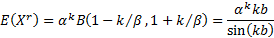Nematrian web functions

Functions relating to the above distribution may be accessed via the Nematrian web function library by using a DistributionName of “loglogistic”. Functions relating to a generalised version of this distribution including an additional location (i.e. shift) parameter may be accessed by using a DistributionName of “loglogistic3”, see also including additional shift and scale parameters. For details of other supported probability distributions see here.